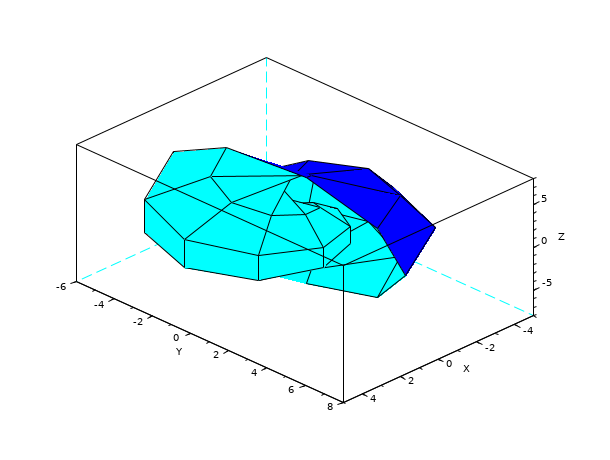Scilab Home page | Wiki | Bug tracker | Forge | Mailing list archives | ATOMS | File exchange
Change language to: Français - Português - 日本語 - Русский
Scilab Help >> Graphics > 3d_plot > eval3dp

eval3dp

compute facets of a 3D parametric surface

Syntax

[Xf,Yf,Zf]=eval3dp(fun,p1,p2)

Arguments

Xf,Yf,Zf

matrices of size (4,n-1*m-1). Xf(:,i) ,Yf(:,i) and Zf(:,i) are respectively the x-axis, y-axis and z-axis coordinates of the 4 points of the ith four sided facet.

fun

a Scilab function.

p1

a vector of size n.

p2

a vector of size m.

Description

eval3dp computes a four sided facets representation of a 3D parametric surface defined by the function fun. fun(p1,p2) computes the x-axis ,y-axis and z-axis coordinates of the corresponding points on the surface, as [x(i),y(i),z(i)]=fun(p1(i),p2(i)). This is used for efficiency.

Examples

p1=linspace(0,2*%pi,10);
p2=linspace(0,2*%pi,10);
deff("[x,y,z]=scp(p1,p2)",["x=p1.*sin(p1).*cos(p2)";..
"y=p1.*cos(p1).*cos(p2)";..
"z=p1.*sin(p2)"])
[Xf,Yf,Zf]=eval3dp(scp,p1,p2);
plot3d(Xf,Yf,Zf)